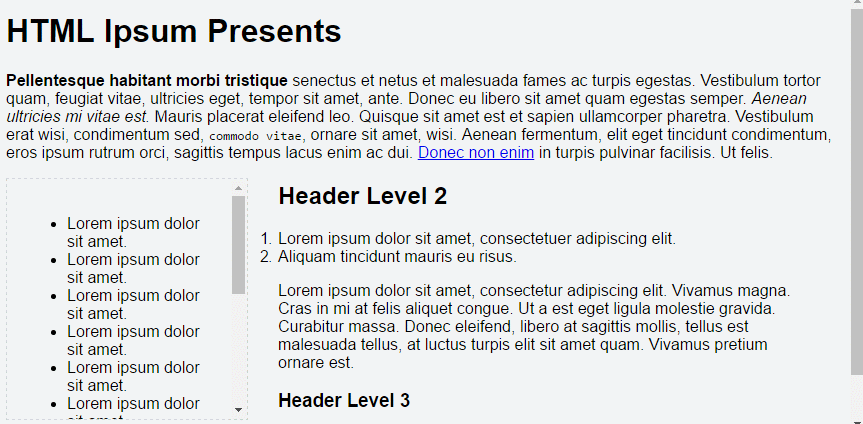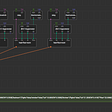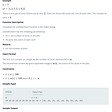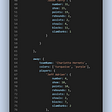# Advent of Code — Day 12 — Javascript

For day 12, you are given a set of direction and and you need to maintain the X and Y position from the origin. It also asks you to keep track of the direction that you are facing. Initially, you are facing east and will be turning in increments of 90 degrees to face a different direction.

`N - Move north x unitsS - Move south x unitsE - Move east x unitsW - Move west x unitsL - Turn left y degreesR - Turn right y degreesF - Move forward based on direction facing`

If it does not make sense yet, let’s take a look at the sample and do a simple walkthrough.

`Initial Direction = [0, 0] and facing EastF10 - Forward 10[10, 0] and facing EastN3[10, 3] and facing EastF7[17, 3] and facing EastR90[17, 3] and facing South F11[17, -8]`

The value that Advent of Code asks for is the sum of the absolute value of the x and y value: 17 + 8 = 25

We start the program as we always do with splitting the directions by newline characters. We will need two variables to keep track of the x and y axis. We also need a variable to keep track of the direction that we are facing.

`function day12(input){    let xAxis = 0    let yAxis = 0    //[North, East, South, West]    let facing = 1    let directions = input.split('\n')}`

We will be iterating through each set of directions and using the first letter to decide which function we will be performing. Each direction follows a pattern of a letter instruction followed by a number.

`function day12(input){    let xAxis = 0    let yAxis = 0    //[North, East, South, West]    let facing = 1    let directions = input.split('\n')    for(let i = 0; i < directions.length; i++){        let instr = directions[i]        letter letter = instr        let num = parseInt(instr.slice(1, instr.length))    }}`

The number in each instruction can be multiple digits which means that we cannot use a split on the whole thing.

`function day12(input){    let xAxis = 0    let yAxis = 0    //[North, East, South, West]    let facing = 1    let directions = input.split('\n')    for(let i = 0; i < directions.length; i++){        let instr = directions[i]        letter letter = instr        let num = parseInt(instr.slice(1, instr.length))        if(instr === "N")             yAxis += num        if(instr === "N")             yAxis += num        if(instr === "N")             yAxis += num        if(instr === "N")             yAxis += num    }}`

We have taken care of the X and Y positioning with this.

The left and right turning is given in increments of 90. When turning left and right

`function day12(input){    let xAxis = 0    let yAxis = 0    //[North, East, South, West]    let facing = 1    let directions = input.split('\n')    for(let i = 0; i < directions.length; i++){        let instr = directions[i]        let letter = instr        let num = parseInt(instr.slice(1, instr.length))        if(instr === "N")             yAxis += num        if(instr === "N")             yAxis += num        if(instr === "N")             yAxis += num        if(instr === "N")             yAxis += num//[North, East, South, West] //Starts East        if(instr === "L"){            facing -= (num / 90)             //L90 = Facing - 1             //L180 = Facing - 2            facing %= 4        }        if(instr === "R"){            facing += (num / 90)            facing %= 4        }        if(instr === "F" && facing === 0)            yAxis += num         if(instr === "F" && facing === 1)            xAxis += num        if(instr === "F" && facing === 2)            yAxis -= num        if(instr === "F" && facing === 3)            xAxis -= num    }    return Math.abs(xAxis) + Math.abs(yAxis)}`

--

--

--

## More from Vincent Yang

Love podcasts or audiobooks? Learn on the go with our new app.

## As busy as a bee — lazy loading in the Angular CLI## ReactJS: Hooks## Improve Web Image Performance with These Tips## jQuery.scrollup.js Plugin## XOD 0.36.0: Records and JSON Serialization## CS373 Fall 2021 Blog #8## Why exactly do we need a Virtual DOM?## SPA Session Timeout — Vue +Rails Implementation## Sales by Match solution in Javascript## Technical Code Series Part 1: Destructuring Objects## Must know principles for an Object Oriented Programmer and an introduction for JavaScript